Question

# For the following reaction, find Q for the conditions Indicated 298 K). If ∆G0 is 20.2...

For the following reaction, find Q for the conditions Indicated 298 K). If ∆G0 is 20.2 kJ/mol, is this reaction at equilibrium?

2 A + B → C

0.25M 0.50M 0.75M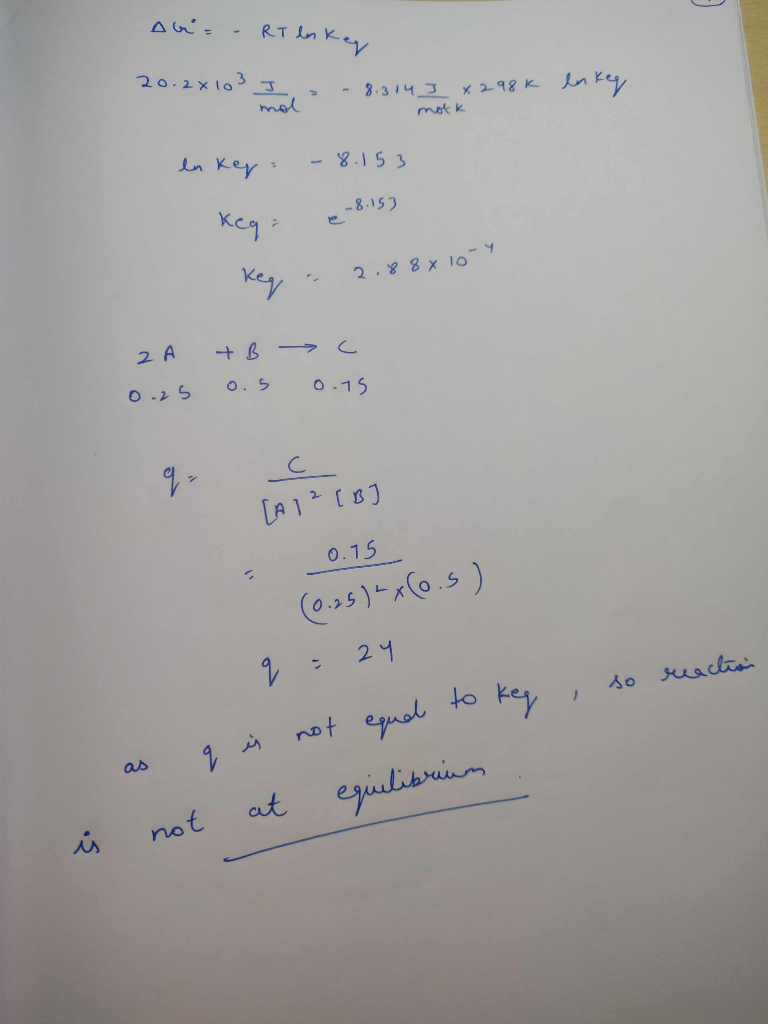#### Earn Coins

Coins can be redeemed for fabulous gifts.

Similar Homework Help Questions
• ### Calculate the equilibrium constant under standard conditions (K’eq) when (delta)G0’ is -4.18 kJ/mol. Standard conditions are...

Calculate the equilibrium constant under standard conditions (K’eq) when (delta)G0’ is -4.18 kJ/mol. Standard conditions are 298 K and a gas constant = 8.32 x 10^-3 kJ/mol K

• ### For a gaseous reaction, standard conditions are 298 K and a partial pressure of 1 atm...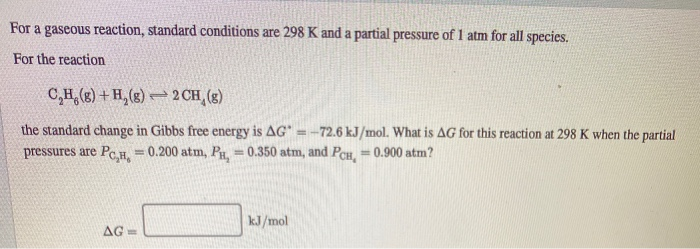For a gaseous reaction, standard conditions are 298 K and a partial pressure of 1 atm for all species. For the reaction C,H,(8) +H,() 2CH (8) the standard change in Gibbs free energy is AG' - -72.6 kJ/mol. What is AG for this reaction at 298 K when the partial pressures are PcH = 0.200 atm, Pr, = 0.350 atm, and PcH, = 0.900 atm? kJ/mol AG=

• ### For a gaseous reaction, standard conditions are 298 K and a partial pressure of 1 atm...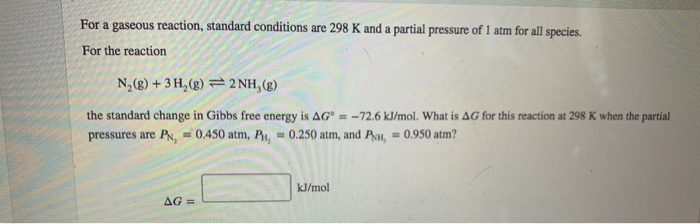For a gaseous reaction, standard conditions are 298 K and a partial pressure of 1 atm for all species. For the reaction Ng(8) + 3 H (8)=2 NH (8) the standard change in Gibbs free energy is AG° = -72.6 kJ/mol. What is AG for this reaction at 298 K when the partial pressures are PN, = 0.450 atm, P, = 0.250 atm, and PH, = 0.950 atm? kJ/mol AG -

• ### For a gaseous reaction, standard conditions are 298 K and a partial pressure of 1 bar...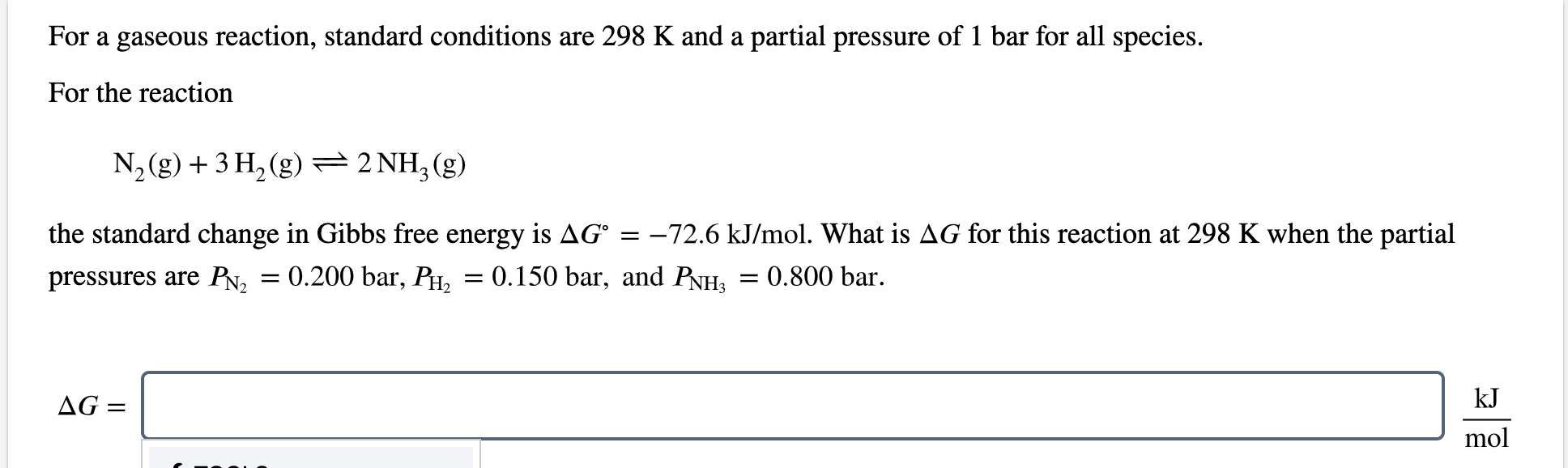For a gaseous reaction, standard conditions are 298 K and a partial pressure of 1 bar for all species. For the reaction N2(g) + 3 H2(g) = 2 NH3(g) the standard change in Gibbs free energy is AGⓇ = -72.6 kJ/mol. What is AG for this reaction at 298 K when the partial pressures are PN, = 0.200 bar, Ph, = 0.150 bar, and PnHz = 0.800 bar. kJ AG = mol

• ### For a gaseous reaction, standard conditions are 298 K and a partial pressure of 1 atm...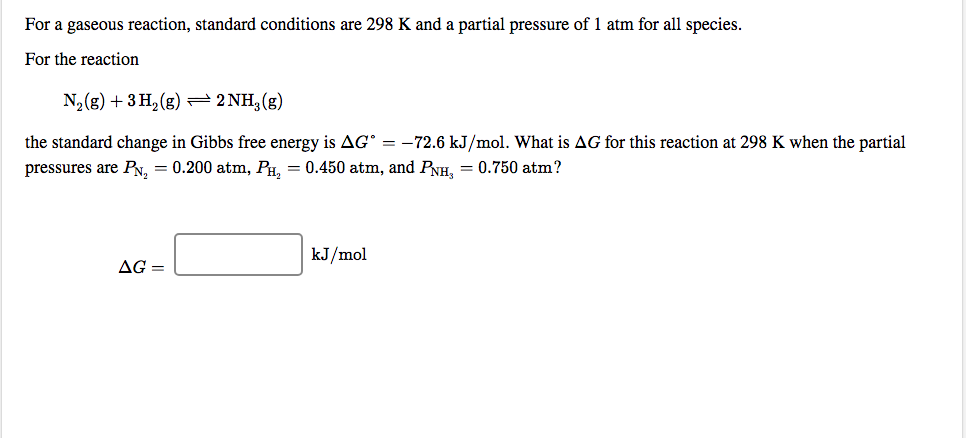For a gaseous reaction, standard conditions are 298 K and a partial pressure of 1 atm for all species. For the reaction N (8) + 3H2(g) + 2NH3(g) the standard change in Gibbs free energy is AGⓇ = -72.6 kJ/mol. What is AG for this reaction at 298 K when the partial pressures are PN, = 0.200 atm, PH, = 0.450 atm, and PNH, = 0.750 atm? AG= L kJ/mol AG= kJ/mol

• ### For a gaseous reaction, standard conditions are 298 K and a partial pressure of 1 atm...

For a gaseous reaction, standard conditions are 298 K and a partial pressure of 1 atm for all species. For the reaction N 2 ( g ) + 3 H 2 ( g ) − ⇀ ↽ − 2 NH 3 ( g ) the standard change in Gibbs free energy is Δ G ° = − 32.8 kJ/mol . What is ΔG for this reaction at 298 K when the partial pressures are P N 2 = 0.100 atm...

• ### Find ΔrG for reaction (in kJ mol-1) at 298 K? 2 NO2 (g) ⇌ N2O4 (g)   Given Conditions:  Pressure:...

Find ΔrG for reaction (in kJ mol-1) at 298 K? 2 NO2 (g) ⇌ N2O4 (g)   Given Conditions:  Pressure: NO2 = 1.25 bar   Pressure: N2O4 = 0.65 bar N2O4(g) --

• ### For a gaseous reaction, standard conditions are 298 K and a partial pressure of 1 atm...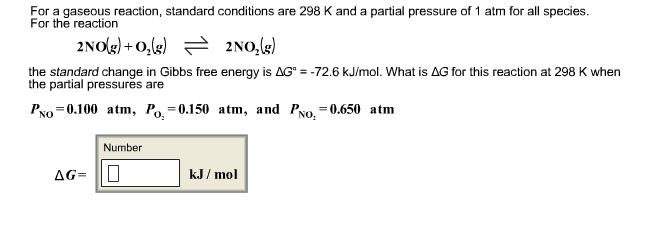For a gaseous reaction, standard conditions are 298 K and a partial pressure of 1 atm for all species. For the reaction the standard change in Gibbs free energy is delta G degree = -72.6 kJ/mol. What is delta G for this reaction at 298 K when the partial pressures are PNO = 0.l00 atm, PO2 = 0.150 atm, and PNO2 = 0.650 atm Number delta G = kJ/mol

• ### For a gascous reaction, standard conditions are 298 K and a partial pressure of 1 atm...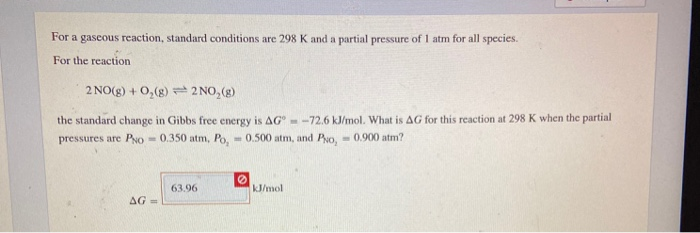For a gascous reaction, standard conditions are 298 K and a partial pressure of 1 atm for all species. For the reaction (2NO(g) +0,(8) 2NO,(8) the standard change in Gibbs free energy is AG - -72.6 kJ/mol. What is AG for this reaction at 298 K when the partial pressures are Pro -0.350 atm, Po, -0.500 atm, and Pro, -0.900 atm? 63.96 kJ/mol AG -

• ### For a gaseous reaction, standard conditions are 298 K and a partial pressure of 1 atm...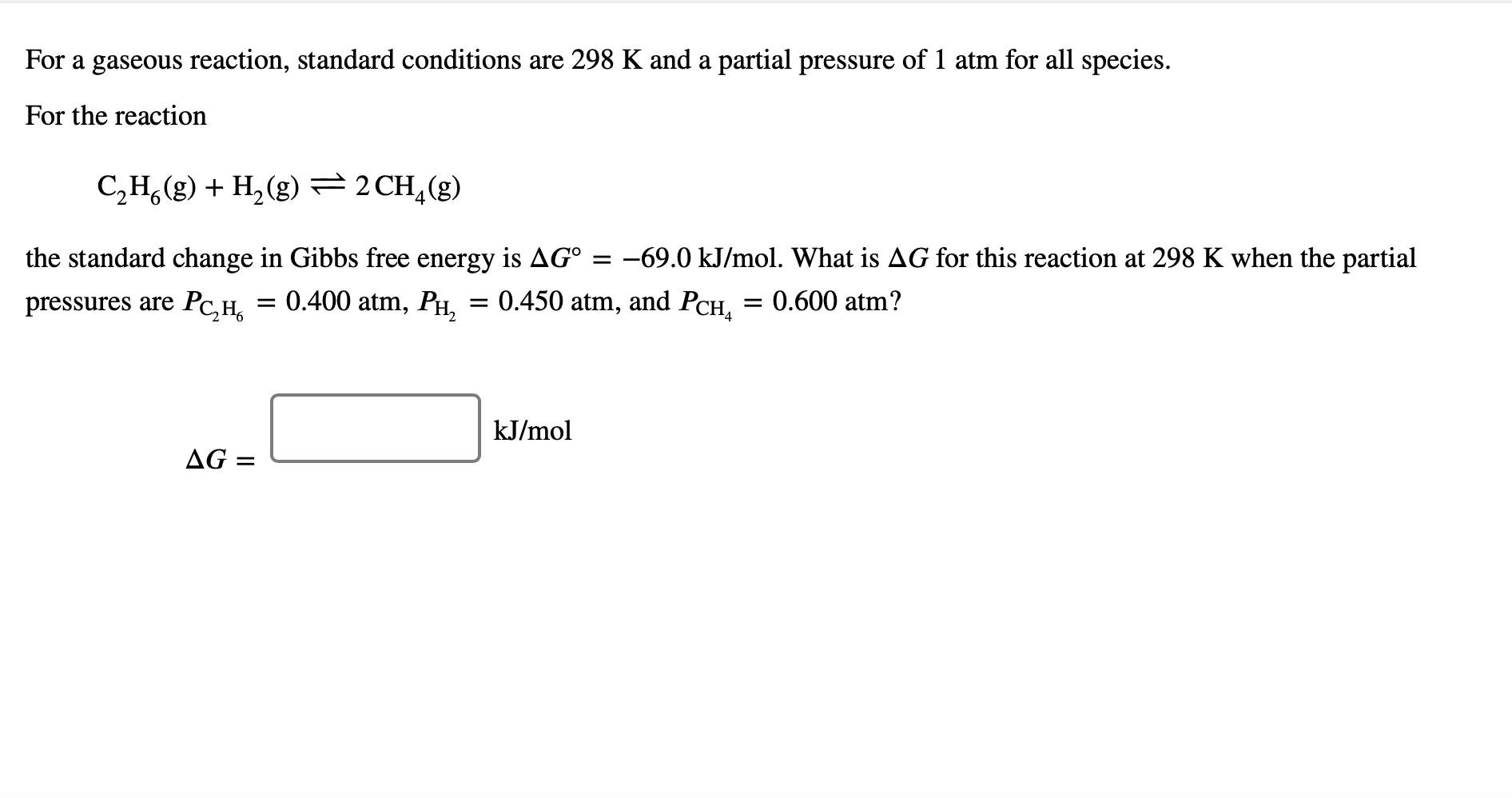For a gaseous reaction, standard conditions are 298 K and a partial pressure of 1 atm for all species. For the reaction C2H(g)H2(g)2 CH (g) 4 the standard change in Gibbs free energy is AG° -69.0 kJ/mo. What is AG for this reaction at 298 K when the partial 0.400 atm, PH Рс,н, 0.450 atm, and PCH, = 0.600 atm? pressures are kJ/mol AG =